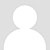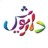# 111111的个人空间

ا=a,aeب=bپ=pت=tث=sج=jچ=chح=hخ=khد=dذ=zر=rز=zژ=zhس=sش=shص=sض=zط=tظ=zع='غ=ghف=fق=qک=kگ=gل=lم=mن=nه=hو=vی=y数字拉丁转写 ۰=صفر(sefr)١=یک(yek)۲=دو...zhyj_y2005请问有波斯语转写拉丁字母（罗马字母）的网站？有波斯语转写国际音标的网站呢？谢谢？我的QQ717608285，邮箱：nxdxxb@126.com谢谢！！期待您的回复(2015-12-29 15:38)dariush波斯语的转写确实是个比较有意思的现象，就连伊朗人自己都没有统一的写法 很多情况下不止一种写法，而同一个字母的转写又是也不是单一的： آ 的转写可以简单写成/a/，因为容易跟短元音/a/混淆，规范一点的可以写成/a:/，也有写成/aa/的； ژ 的发音可以写成/jh/，其实发音更接...(2011-08-09 21:51)